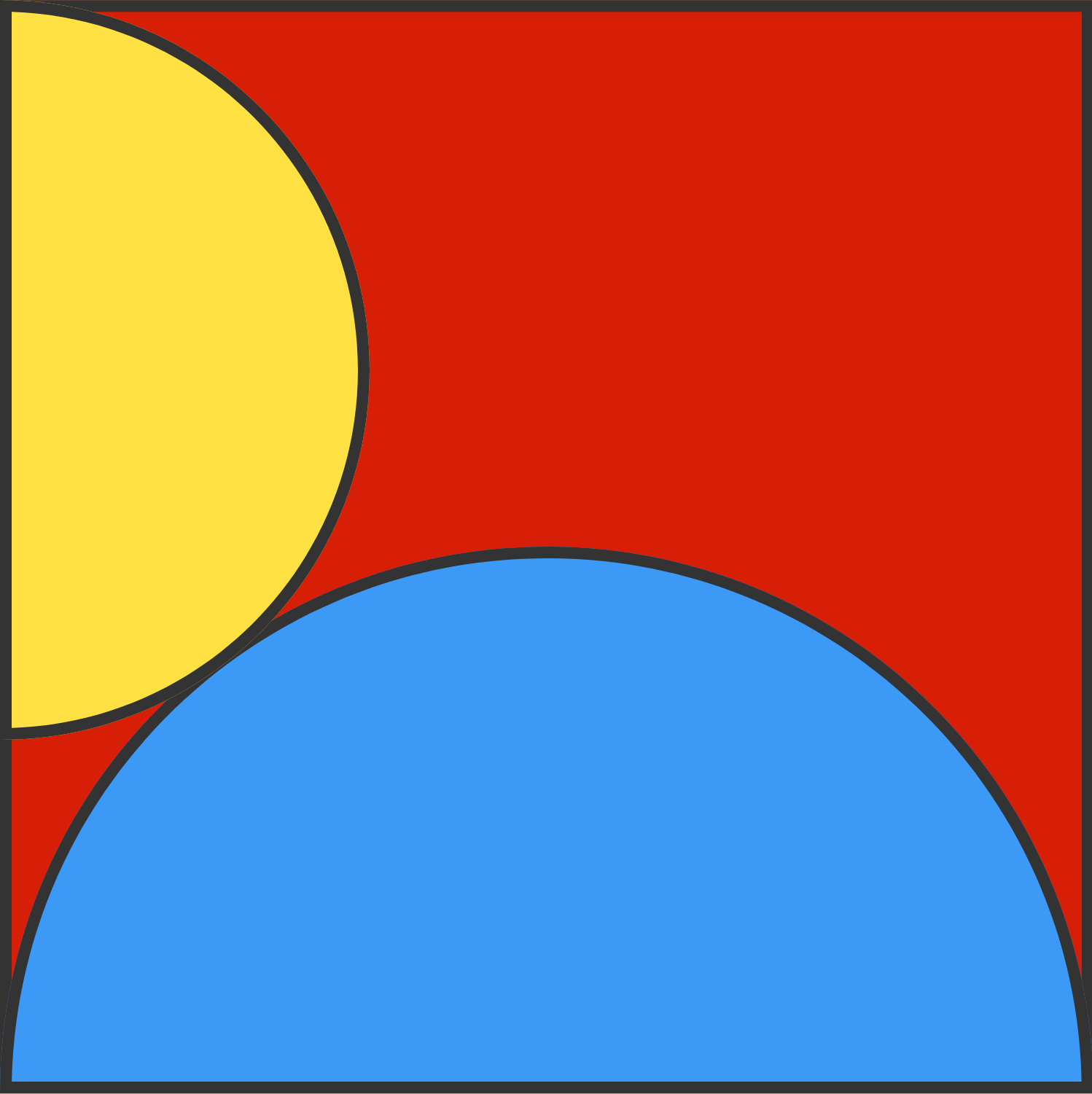# SemicirclesIn the image above there are two tangent semicircles. The side length of the square is 36cm.

What is the length of the radius of the yellow circle? Give your answer in $\text{cm}$.

×